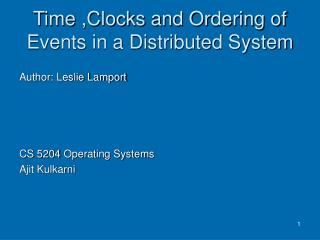# Time ,Clocks and Ordering of Events in a Distributed System - PowerPoint PPT PresentationDownload PresentationTime ,Clocks and Ordering of Events in a Distributed System

Time ,Clocks and Ordering of Events in a Distributed System
Download Presentation## Time ,Clocks and Ordering of Events in a Distributed System

- - - - - - - - - - - - - - - - - - - - - - - - - - - E N D - - - - - - - - - - - - - - - - - - - - - - - - - - -
##### Presentation Transcript

1. Time ,Clocks and Ordering of Events in a Distributed System Author: Leslie Lamport CS 5204 Operating Systems Ajit Kulkarni

2. Need for Clocks • Synchronization in Distributed Systems • Utilize timers • implementation? • accuracy? • Do we need timers? • ‘what happened before what?’

3. History Behind Paper • The Maintenance of Duplicate Databases by Paul Johnson and Bob Thomas • Use of timestamps • Incorrect algorithm

4. Special Relativity • No invariant total ordering of events in space-time • Different observers can disagree about which of two events happened first • Partial order in which an event e1 precedes an event e2e1 can causally affect e2

5. Partial Ordering in Computer Systems • Uniprocess a and b , events in the same process, and a comes before b, then a  b • Multiprocess First process sends message :event a ,second process receives it :eventb then a  b • Transitivity If a b and b  c then a c

6. Goals of Paper • Not to rely on global time • Order events based on logical clocks

7. Special cases of Ordering • Concurrent Events • Independent input and output • No causal order • Happens before relation • Events a and ba->b implies a happens before b • a can causally affect b

8. Process i Process k Process j Example (I) d a X X c e X X b X

9. Results • From first condition • ad • ce • From second condition • ac • be • From third condition • ae

10. Do we need clocks? • Two independent events…NO • Two dependent events …YES but • Can we do better than timers

11. Logical Clocks • A counter attached to each process • Counter updates after every new event • Achieves partial ordering • System of Clocks: Function C which assigns to any event b the number C(b), where C(b) = Cj(b)

12. Clock Condition • For any events a,b :if ab then C(a) < C(b) • Satisfying conditions: • If Ci(a)<Ci(b) implies a comes beforeb • Ci(a) < Cj(b) implies b received message from a where i, j are different

13. System of clocks satisfying conditions • Process increments counter between two successive events • Between 2 processes • Process sends message with a timestamp • On receiving message process sets counter to value greater (its timestamp ,value in message)

14. Total Ordering • One common order for everyone • A relation ==>,a ==>b if and only if either • Ci(a) < Ci(b) or • Ci(a) = Cj(b) and Pi < Pj • If ab then a==>b

15. Ordering of events

16. Anomalous Behaviors • Seen in logical clocks with external interactions

17. Process i Process k Process j Example (II) a X c e X X b d X X

18. Results • Process i sends message to process k a->d • Process i sends external message to process j a->c • Process i sends message to process k c->b • Resource granted to process j

19. Strong Clock Condition • For any events a,b in S if ab then C(a) < C(b) where S is set of all system events and external events

20. Mutual exclusion example • Process granted the resource must release it before it can be granted to another process • Different requests for the resource must be granted in the order in which they are made • If every process granted the resource eventually releases it, then every request is eventually granted

21. Physical Time • C i(t) =>clock Ci at physical time t • Conditions for being a true physical clock • derivative is 1 • all clocks nearly same

22. Central Ideas • A precise characterization of causality in distributed systems • a framework for explaining and reasoning about event ordering in distributed protocols

23. Further Research Led by Paper • Vector and matrix clock abstractions • Stable and non stable predicate detection • Shows distributed systems as fundamentally different from other concurrent systems • Byzantine agreement • Byzantine clock synchronization and ordered multicast protocols